# JP6020711B2 - Capacitive sensor and method for correcting nonlinear output - Google Patents

## Info

Publication number
JP6020711B2
JP6020711B2 JP2015510094A JP2015510094A JP6020711B2 JP 6020711 B2 JP6020711 B2 JP 6020711B2 JP 2015510094 A JP2015510094 A JP 2015510094A JP 2015510094 A JP2015510094 A JP 2015510094A JP 6020711 B2 JP6020711 B2 JP 6020711B2
Authority
JP
Japan
Prior art keywords
acceleration
movable electrode
capacitive sensor
correction
electrode
Prior art date
Legal status (The legal status is an assumption and is not a legal conclusion. Google has not performed a legal analysis and makes no representation as to the accuracy of the status listed.)
Active
Application number
JP2015510094A
Other languages
Japanese (ja)
Other versions
JPWO2014163076A1 (en
Inventor

Original Assignee

Priority date (The priority date is an assumption and is not a legal conclusion. Google has not performed a legal analysis and makes no representation as to the accuracy of the date listed.)
Filing date
Publication date
Priority to JP2013077145 priority Critical
Priority to JP2013077145 priority
Application filed by 富士電機株式会社 filed Critical 富士電機株式会社
Priority to PCT/JP2014/059634 priority patent/WO2014163076A1/en
Application granted granted Critical
Publication of JP6020711B2 publication Critical patent/JP6020711B2/en
Publication of JPWO2014163076A1 publication Critical patent/JPWO2014163076A1/en
Application status is Active legal-status Critical
Anticipated expiration legal-status Critical

## Images

•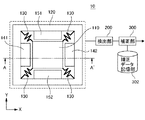•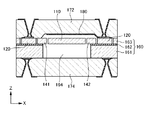•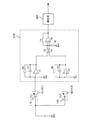•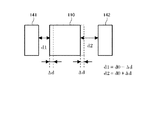•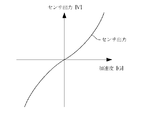•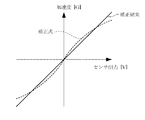•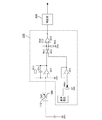•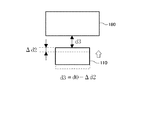•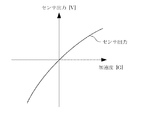•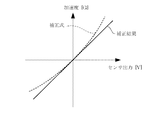•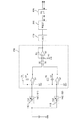•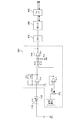•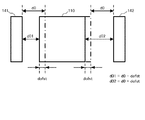•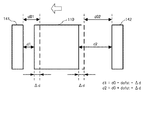••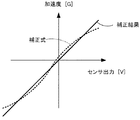•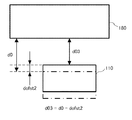•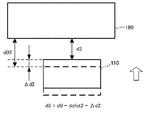•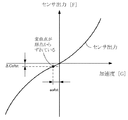•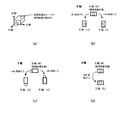## Classifications

• GPHYSICS
• G01MEASURING; TESTING
• G01RMEASURING ELECTRIC VARIABLES; MEASURING MAGNETIC VARIABLES
• G01R35/00Testing or calibrating of apparatus covered by the preceding groups
• G01R35/005Calibrating; Standards or reference devices, e.g. voltage or resistance standards, "golden" references
• GPHYSICS
• G01MEASURING; TESTING
• G01PMEASURING LINEAR OR ANGULAR SPEED, ACCELERATION, DECELERATION, OR SHOCK; INDICATING PRESENCE, ABSENCE, OR DIRECTION, OF MOVEMENT
• G01P15/00Measuring acceleration; Measuring deceleration; Measuring shock, i.e. sudden change of acceleration
• G01P15/02Measuring acceleration; Measuring deceleration; Measuring shock, i.e. sudden change of acceleration by making use of inertia forces using solid seismic masses
• G01P15/08Measuring acceleration; Measuring deceleration; Measuring shock, i.e. sudden change of acceleration by making use of inertia forces using solid seismic masses with conversion into electric or magnetic values
• G01P15/125Measuring acceleration; Measuring deceleration; Measuring shock, i.e. sudden change of acceleration by making use of inertia forces using solid seismic masses with conversion into electric or magnetic values by capacitive pick-up
• GPHYSICS
• G01MEASURING; TESTING
• G01PMEASURING LINEAR OR ANGULAR SPEED, ACCELERATION, DECELERATION, OR SHOCK; INDICATING PRESENCE, ABSENCE, OR DIRECTION, OF MOVEMENT
• G01P15/00Measuring acceleration; Measuring deceleration; Measuring shock, i.e. sudden change of acceleration
• G01P15/18Measuring acceleration; Measuring deceleration; Measuring shock, i.e. sudden change of acceleration in two or more dimensions
• GPHYSICS
• G01MEASURING; TESTING
• G01PMEASURING LINEAR OR ANGULAR SPEED, ACCELERATION, DECELERATION, OR SHOCK; INDICATING PRESENCE, ABSENCE, OR DIRECTION, OF MOVEMENT
• G01P21/00Testing or calibrating of apparatus or devices covered by the preceding groups
• GPHYSICS
• G01MEASURING; TESTING
• G01RMEASURING ELECTRIC VARIABLES; MEASURING MAGNETIC VARIABLES
• G01R27/00Arrangements for measuring resistance, reactance, impedance, or electric characteristics derived therefrom
• G01R27/02Measuring real or complex resistance, reactance, impedance, or other two-pole characteristics derived therefrom, e.g. time constant
• G01R27/26Measuring inductance or capacitance; Measuring quality factor, e.g. by using the resonance method; Measuring loss factor; Measuring dielectric constants ; Measuring impedance or related variables
• G01R27/2605Measuring capacitance

## Description

The present invention relates to a capacitance type sensor that detects acceleration and a correction method for nonlinear output.

As an acceleration detection device for detecting acceleration, for example, as described in Patent Document 1, there is a capacitance type device. This capacitance-type device detects acceleration by arranging a fixed electrode in the vicinity of the movable electrode and detecting a change in capacitance between the movable electrode and the fixed electrode.

Patent Documents 1, 2, and 3 show that the relationship between the change in capacitance and the amount of displacement of the movable electrode is non-linear. In Patent Documents 1, 2, and 3, it is described that since the displacement amount of the movable electrode is very small, the relationship between the change in capacitance and the displacement amount of the movable electrode can be regarded as linear.

The acceleration sensors disclosed in Patent Documents 4 and 5 regard the relationship between the change in capacitance and the displacement amount of the movable electrode as non-linear, and correct this non-linearity. The acceleration sensor disclosed in Patent Document 4 is a cylindrical three-axis acceleration sensor. This acceleration sensor detects acceleration for each of the three axes based on a change in capacitance between electrodes on the fixed disk and electrodes on the movable disk facing each other in the extending direction of the cylinder. The acceleration sensor of Patent Document 5 detects the acceleration in one direction.

Japanese Patent Laid-Open No. 2-110383 Japanese Patent Laid-Open No. 5-72225 JP-A-5-340958 JP-A-8-313522 Japanese Patent Laid-Open No. 7-120498

The present inventor studied the construction of a capacitive sensor having a movable electrode and a plurality of fixed electrodes opposed to the movable electrode from different directions. The inventor has studied a method of accurately detecting acceleration in each of a plurality of directions in this capacitive sensor.

The present invention has been made in view of the above circumstances, and an object thereof is to provide a movable electrode and a plurality of fixed electrodes facing the movable electrode from different directions, respectively, in a plurality of directions. It is an object of the present invention to provide a capacitance type sensor and a correction method capable of accurately detecting acceleration.

The capacitance type sensor according to the present invention includes a movable electrode, a support, a beam member, a first fixed electrode, a second fixed electrode, a detection unit, and a correction unit. The beam member is attached in a state where the movable electrode is movable to the support. The first fixed electrode is opposed to the movable electrode from the first direction. The second fixed electrode is opposed to the movable electrode from a second direction different from the first direction. A detection part detects the change of the electrostatic capacitance which arises between a movable electrode and each fixed electrode. The correction unit corrects the detection result of the detection unit and generates an acceleration signal indicating the acceleration. There are a plurality of combinations of fixed electrodes and movable electrodes. A correction | amendment part correct | amends for every kind of combination based on a mutually different correction method.

The nonlinear output correction method according to the present invention is a method of correcting the output of the detection unit in the above-described capacitance type sensor . Using the spring constant of the distance and the beam member between the variable dynamic and fixed electrodes, the theoretical expression for calculating the electrostatic capacitance of the acceleration is calculated. And the output of a detection part is correct | amended using the inverse transformation type | formula of a theoretical formula.

According to the present invention, acceleration can be accurately detected in each of a plurality of directions in a capacitive sensor having a movable electrode and a plurality of fixed electrodes facing the movable electrode from different directions.

The above-described object and other objects, features, and advantages will become more apparent from the preferred embodiments described below and the accompanying drawings.

It is a top view which shows the structure of the electrostatic capacitance type sensor which concerns on 1st Embodiment. It is AA 'sectional drawing of FIG. It is a figure which shows the circuit structure of the detection part of an electrostatic capacitance type sensor. It is a figure which shows a motion of a movable electrode when the acceleration of the X direction in FIGS. 1, 2 is added to the movable electrode of an electrostatic capacitance type sensor. It is a figure which shows the relationship between the output from a detection part, and the acceleration G. FIG. It is a figure which shows the output (1st acceleration signal) after correct | amending the correction formula shown to Formula (6), and output (DELTA) C shown in FIG. 5 with this correction formula. It is a figure which shows the circuit structure of the detection part of an electrostatic capacitance type sensor. It is a figure which shows a motion of a movable electrode when the acceleration of the Z direction in FIG. 2 is added to the movable electrode of an electrostatic capacitance type sensor. It is a figure which shows the relationship between the output from a detection part, and acceleration G. FIG. It is a figure which shows the output (2nd acceleration signal) after correct | amending the correction formula shown to Formula (11), and the output shown in FIG. 9 with this correction formula. It is a figure which shows the function structure of the capacitive sensor which concerns on 2nd Embodiment. It is a figure which shows the function structure of the capacitive sensor which concerns on 2nd Embodiment. It is a figure which shows the positional relationship of the fixed electrode and movable electrode in an initial state about the X direction in FIGS. It is a figure which shows a motion of a movable electrode when the acceleration of the X direction in FIG.1, 2 is added to the movable electrode of an electrostatic capacitance type sensor. It is a figure which shows the relationship between the output from the detection part and the acceleration G in 3rd Embodiment. It is a figure which shows the output (1st acceleration signal) after correct | amending the correction formula shown to Formula (19), and output (DELTA) C shown in FIG. 15 by this correction formula. It is a figure which shows the positional relationship of the movable electrode and fixed electrode in an initial state about the Z direction in FIG. 2 of an electrostatic capacitance type sensor. It is a figure which shows a motion of a movable electrode when the acceleration of the Z direction in FIG. 2 is added to the movable electrode of an electrostatic capacitance type sensor. FIG. 14 is a diagram showing a relationship between an output ΔC and an acceleration G when the initial deviation d ofst ≠ 0 in FIG. 13. It is a figure showing the range of the acceleration which can be applied with respect to the housing | casing of an electrostatic capacitance type sensor using a gravitational acceleration.

Hereinafter, embodiments of the present invention will be described with reference to the drawings. In all the drawings, the same reference numerals are given to the same components, and the description will be omitted as appropriate.

In the following description, each component of the detection unit 200 and the correction unit 300 is not a hardware unit configuration but a functional unit block. The detection unit 200 and the correction unit 300 are an arbitrary computer CPU, memory, a program loaded in the memory, a storage medium such as a hard disk for storing the program, and an interface for network connection. Realized by combination. There are various modifications to the implementation method.

(First embodiment)
FIG. 1 is a plan view showing the configuration of the capacitive sensor 10 according to the first embodiment. 2 is a cross-sectional view taken along the line AA ′ of FIG. The capacitive sensor 10 according to this embodiment includes a movable electrode 110, a support 120, a beam member 130, fixed electrodes 141 and 142, a detection unit 200, and a correction unit 300. The beam member 130 functions as a spring, and the movable electrode 110 is attached to the support 120 in a movable state. The detection unit 200 detects a change in capacitance that occurs between the movable electrode 110 and the fixed electrodes 141 and 142. Then, the correction unit 300 corrects the detection result of the detection unit 200 and generates an acceleration signal indicating the acceleration.

According to the present embodiment, the acceleration signal has a value obtained by correcting the detection result of the detection unit 200. For this reason, the relationship between the change in capacitance and the acceleration signal can be made nonlinear. Therefore, the acceleration can be detected with high accuracy. Hereinafter, the capacitive sensor 10 will be described in detail.

The movable electrode 110 has a flat plate shape, and its planar shape is a rectangle. The fixed electrode 141 (first fixed electrode) is opposed to the first side (edge) of the movable electrode 110, and the fixed electrode is disposed on the side of the movable electrode 110 facing the first side (second side). 142 (second fixed electrode) faces each other. The fixed electrodes 141 and 142 are opposed to each other via the movable electrode 110, and are disposed at positions that are symmetrical with respect to each other with respect to a line that passes through the center of the movable electrode 110 and is parallel to the first side. .

In the example shown in the figure, each side of the movable electrode 110 is linear. However, these sides may be comb-like. In this case, the sides of the fixed electrodes 141 and 142 facing the movable electrode 110 are also comb-like and are arranged so as to engage with the movable electrode 110.

The support 120 is provided so as to surround the four sides of the movable electrode 110. Specifically, the support 120 has a rectangular opening. The four sides of the opening are parallel to the four sides of the movable electrode 110. Four beam members 130 are provided. One end of each beam member 130 is attached to the corner of the movable electrode 110, and the other end is attached to the corner of the opening of the support 120. However, the arrangement of the beam members 130 is not limited to the example shown in this figure.

The capacitive sensor 10 includes fixed electrodes 151 and 152. The fixed electrode 151 faces the third side of the movable electrode 110, and the fixed electrode 152 faces the side of the movable electrode 110 that faces the third side (fourth side).

In FIG. 2, the capacitive sensor 10 is formed using an SOI (Silicon On Insulator) substrate 160. This SOI substrate 160 includes a lower silicon support layer 161, a silicon oxide layer 162 formed on the silicon support layer 161, and an active silicon layer 163 formed on the silicon oxide layer 162. . The silicon support layer 161 and the silicon oxide layer 162 are removed by etching, leaving portions (supports 120) surrounding the four sides of the movable electrode 110. In this way, the space portion 164 is formed in the center portion of the silicon support layer 161 and the silicon oxide layer 162.

On the other hand, the upper active silicon layer 163 is partially removed by, for example, anisotropic dry etching. By this removal, the movable electrode 110 located at the center from the active silicon layer 163, the beam member 130 (elastic member) extending outward from the four corners diagonally from the four corners of the movable electrode 110, and four of the movable electrode 110. Fixed electrodes 141, 142, 151, and 152 are formed to face the sides with a predetermined interval.

Further, the capacitive sensor 10 has protective members 172 and 174. The protection members 172 and 174 are formed using glass, for example. The protection member 172 covers the surface (front surface) of the substrate 160 where the movable electrode 110 is formed, and the protection member 174 covers the back surface of the substrate 160. The protection members 172 and 174 are separated from the movable electrode 110 and the like except for the edges. A fixed electrode 180 (third fixed electrode) is formed on the surface of the protective member 172 facing the movable electrode 110. That is, the fixed electrode 180 faces the movable electrode 110 from a different direction from the fixed electrodes 141, 142, 151, and 152. In other words, the fixed electrodes 141 and 142 are opposed to the movable electrode 110 from the first direction (X direction in FIGS. 1 and 2), and the fixed electrode 180 is movable from the second direction (Z direction in FIG. 2). Opposite the electrode 110. The second direction is a direction facing the front surface or the back surface of the movable electrode 110.

Then, the correction unit 300 changes the electrostatic capacity (first electrostatic capacity) between the movable electrode 110 and the fixed electrode 141 and the electrostatic capacity (second electrostatic capacity) between the movable electrode 110 and the fixed electrode 142. ), The component perpendicular to the first side of the movable electrode 110 (the component in the X direction in FIGS. 1 and 2: the acceleration in the first direction) is detected from the acceleration applied to the movable electrode 110 and detected. A first acceleration signal indicating the magnitude of acceleration is output. Specifically, the capacitance between the movable electrode 110 and the fixed electrode 141 changes in the opposite direction to the capacitance between the movable electrode 110 and the fixed electrode 142. For this reason, the structure which detects the component perpendicular | vertical to the 1st edge | side of the movable electrode 110 among the accelerations applied to the movable electrode 110 among the capacitive sensors 10 has a differential structure symmetrical in the X direction. .

Further, the correction unit 300 determines the acceleration applied to the movable electrode 110 based on the change in capacitance between the movable electrode 110 and the fixed electrode 151 and the change in capacitance between the movable electrode 110 and the fixed electrode 152. Among these, a component parallel to the first side of the movable electrode 110 (component in the Y direction in FIG. 1) is detected, and an acceleration signal indicating the magnitude of the detected acceleration is output. The capacitive sensor 10 has a differential structure that is symmetric in the Y direction when detecting a component parallel to the first side of the movable electrode 110 out of the acceleration applied to the movable electrode 110.

In addition, the correction unit 300 generates a component perpendicular to the movable electrode 110 (acceleration applied to the movable electrode 110 based on a change in capacitance (third capacitance) between the movable electrode 110 and the fixed electrode 180). 2), and a second acceleration signal indicating the magnitude of the detected acceleration is output. As described above, the capacitive sensor 10 has an asymmetric structure in the Z direction when detecting a component perpendicular to the movable electrode 110 out of the acceleration applied to the movable electrode 110.

The correction method used by the correction unit 300 differs depending on the combination of the movable electrode 110 and the fixed electrode. Specifically, the correction method used when detecting the component perpendicular to the movable electrode 110 (the component in the Z direction in FIG. 2) among the accelerations applied to the movable electrode 110 is movable among the accelerations applied to the movable electrode 110. This is different from the correction method used when detecting a component perpendicular to the first side of the electrode 110 (component in the X direction in FIGS. 1 and 2).

These correction methods are set as follows, for example. First, using the distance between the movable electrode and the fixed electrode and the spring constant of the beam member, a theoretical formula for calculating the capacitance from the acceleration is calculated. Then, the correction method is set by using the inverse transformation formula of the theoretical formula.

The correction method used by the correction unit 300 is stored in the correction data storage unit 302. The correction data storage unit 302 stores, for example, a correction table. Then, the correction unit 300 generates an acceleration signal based on a correction formula using this correction table. For example, the correction unit 300 calculates a broken line as an approximate expression of the correction expression by connecting two adjacent points among a plurality of points stored in the correction table with a straight line. In this way, the calculation load applied to the correction unit 300 can be reduced.

FIG. 3 is a diagram illustrating a circuit configuration of the detection unit 210 included in the detection unit 200 of the capacitance type sensor 10. The detection unit 210 is connected to the fixed electrodes 141 and 142. In addition, the detection unit 200 includes a circuit connected to the fixed electrodes 151 and 152 separately from the detection unit 210. This circuit also has the same configuration as that of the detection unit 210.

A DC voltage is applied to the movable electrode 110. The fixed electrode 141 is connected to the negative input terminal of the differential amplifier Q1. The positive input terminal of the differential amplifier Q1 is grounded. A resistor R1 and a capacitor C1 are connected in parallel to the negative input terminal of the differential amplifier Q1 and the output terminal of the differential amplifier Q1.

The fixed electrode 142 is connected to the negative input terminal of the differential amplifier Q2. The positive input terminal of the differential amplifier Q2 is grounded. A resistor R2 and a capacitor C2 are connected in parallel to the negative input terminal of the differential amplifier Q2 and the output terminal of the differential amplifier Q2.

The output terminal of the differential amplifier Q1 is input to one input terminal (for example, the negative side input terminal) of the differential amplifier Q3 via the resistor R3, and the output terminal of the differential amplifier Q2 is input via the resistor R4. Is input to the other input terminal (for example, the positive input terminal) of the differential amplifier Q3. A resistor R5 is connected in parallel to one input terminal of the differential amplifier Q3 and the output terminal of the differential amplifier Q3. The resistor R4 and the other input terminal of the differential amplifier Q3 are grounded via a resistor R6. The output of the differential amplifier Q3 is input to the correction unit 300.

The circuits of the differential amplifiers Q1 and Q2 are charge / voltage conversion circuits that convert the capacitance between the connected electrodes into a voltage signal and output the voltage signal. Similarly, the circuit of the differential amplifier Q3 is a difference calculation circuit, and outputs the difference result of (Q2-Q1).

In the calculation of the theoretical formula described later, the output is expressed as capacitance [F] for ease of expression. However, in the actual capacitive sensor 10, the voltage signal [V] converted by the Q1 and Q2 is output.

FIG. 4 shows the movement of the movable electrode 110 when acceleration in the X direction in FIGS. 1 and 2 is applied to the movable electrode 110 of the capacitive sensor 10. The initial distance between the movable electrode 110 fixed electrodes 141 and 142 (electrode interval when the acceleration 0), and d 0. When the movable electrode 110 moves to the left by Δd, the distance d 1 between the movable electrode 110 and the fixed electrode 141 is (d 0 −Δd), and the distance d 2 between the movable electrode 110 and the fixed electrode 142 is (d 0 + Δd).

Then, the capacitance C 1 between the movable electrode 110 and the fixed electrode 141 is obtained by Equation (1).
Here, ε is a dielectric constant, and S 1 is an area of a portion where the movable electrode 110 and the fixed electrode 141 are opposed to each other.

Similarly, the capacitance C 2 between the movable electrode 110 and the fixed electrode 142 is obtained by Equation (2).
Here, S 2 is the area of a portion fixed electrode 142 and the movable electrode 110 is opposed.

Then, S 2 is equal to S 1. For this reason, since the theoretical formula indicating the output ΔC from the detection unit 210 in FIG. 3 is the difference between the formula (1) and the formula (2), the following formula (3) is obtained.

When the mass of the movable electrode 110 is m and the spring constant when the four beam members 130 are regarded as one spring is k, this equation (3) is expressed as f = m · a = k · Δd. By using, it is deformed as follows. This formula is a theoretical formula for calculating the acceleration a.

Here, when α XY : εS 1 · 2 (k / m) and β XY : (k / m) 2 · d 0 2 , Equation (4) becomes the following Equation (5).

And the inverse transformation formula of the above-mentioned formula (5) becomes as the following formula (6).

The correction unit 300 performs correction based on the equation (6) and calculates the first acceleration signal. The specific values of the coefficients α XY and β XY in the equation (6) are as follows: the area of each electrode of the design value of the capacitive sensor 10, the initial spacing d 0 between the electrodes, the dielectric constant between the electrodes, and the weight It can be obtained by using numerical values such as mass and beam spring constant. Specific values of the coefficients α XY and β XY include the area of each electrode of the capacitive sensor 10, the initial distance d 0 between the electrodes, the dielectric constant between the electrodes, the mass of the weight, the spring constant of the beam, etc. A numerical value obtained as a result of actual measurement may be used. In addition, this specific numerical value is obtained by applying a predetermined acceleration (preferably a plurality of accelerations) to each capacitance type sensor 10 and calculating an actual measurement value of the output from the capacitance type sensor 10 at that time (6) It is also possible to calculate by fitting. For the fitting at this time, for example, a least square method can be used. However, the fitting is not limited to the least square method. Data indicating the coefficients α XY and β XY is stored in the correction data storage unit 302. The data stored in the correction data storage unit 302 can be rewritten.

FIG. 5 is a diagram illustrating the relationship between the output ΔC from the detection unit 210 and the acceleration G. As shown in the figure, the relationship between the output ΔC from the detection unit 210 and the acceleration G is non-linear.

FIG. 6 is a diagram showing a correction formula shown in Formula (6) and an output (first acceleration signal) after the output ΔC shown in FIG. 5 is corrected by this correction formula. As shown in the figure, by performing correction by the correction unit 300, the relationship between the output of the correction unit 300 and the acceleration G can be made linear.

In the example shown in the figure, the correction unit 300 may be provided with different correction methods depending on whether the acceleration is positive or negative. For example, different correction methods are set by performing the above-mentioned fitting separately when the acceleration is positive and when the acceleration is negative. In this way, the accuracy of correction by the correction unit 300 increases.

Even when the acceleration in the Y direction in FIG. 1 is applied to the movable electrode 110 of the capacitive sensor 10, the correction unit 300 performs the same correction as described with reference to FIG.

FIG. 7 is a diagram illustrating a circuit configuration of the detection unit 220 included in the detection unit 200 of the capacitance type sensor 10. The detection unit 220 is connected to the fixed electrode 180.

As described above, a DC voltage is applied to the movable electrode 110. The fixed electrode 180 is connected to the negative input terminal of the differential amplifier Q11. The positive input terminal of the differential amplifier Q11 is grounded. A resistor R11 and a capacitor C11 are connected in parallel to the negative input terminal of the differential amplifier Q11 and the output terminal of the differential amplifier Q11.

The output terminal of the differential amplifier Q11 is input to one input terminal (for example, negative input terminal) of the differential amplifier Q13 via the resistor R12. The output terminal of the differential amplifier Q12 is connected to the other input terminal (for example, the positive input terminal) of the differential amplifier Q13 via the resistor R13. One input terminal (eg, negative input terminal) of the differential amplifier Q12 is connected to the output terminal of the differential amplifier Q12, and the other input terminal (eg, positive input terminal) of the differential amplifier Q12 is connected to the differential amplifier Q12. The reference voltage is applied via the variable resistor VR11.

A resistor R14 is connected in parallel to one input terminal of the differential amplifier Q13 and the output terminal of the differential amplifier Q13. The resistor R13 and the other input terminal of the differential amplifier Q13 are grounded via a resistor R15. The output of the differential amplifier Q13 is input to the correction unit 300.

FIG. 8 shows the movement of the movable electrode 110 when acceleration in the Z direction in FIG. 2 is applied to the movable electrode 110 of the capacitive sensor 10. The initial interval between the movable electrode 110 and the fixed electrode 180 (the electrode interval when the acceleration is 0) is d 0 . When the movable electrode 110 moves upward by Δd 2 , the distance d 3 between the movable electrode 110 and the fixed electrode 180 becomes (d 0 −Δd 2 ).

Then, the capacitance C 3 between the fixed electrode 180 and the movable electrode 110 is obtained by Equation (7).
Here, ε is a dielectric constant, and S 3 is an area of a portion where the movable electrode 110 and the fixed electrode 180 are opposed to each other.

Then, the theoretical expression indicating the output ΔC from the detection unit 220 in FIG. 7 is as the following expression (8).

When the mass of the movable electrode 110 is m, and the spring constant in the Z direction when the four beam members 130 are regarded as one spring is k 2 , f = m · a = k 2 · Δd 2 is used. Equation (8) can be transformed into Equation (9). This equation (9) is a theoretical equation for calculating the acceleration a.

Here, when α z : ε · S 3 · (1 / d 0 ) and β z : (k 2 / m) · d 0 , the equation (9) becomes the following equation (10).

And the inverse transformation formula of the above-mentioned formula (10) is as the following formula (11).

The correcting unit 300 performs correction based on the equation (11) and calculates the second acceleration signal. Then, specific numerical values of the coefficients α z and β z in the equation (11) can be obtained by the same method as the above-described coefficients α xy and β xy .

FIG. 9 is a diagram illustrating the relationship between the output ΔC from the detection unit 220 and the acceleration G. As shown in the figure, the relationship between the output ΔC from the detection unit 220 and the acceleration G is non-linear.

FIG. 10 is a diagram showing a correction formula shown in Formula (11) and an output (second acceleration signal) after the output ΔC shown in FIG. 9 is corrected by this correction formula. As shown in the figure, by performing correction by the correction unit 300, the relationship between the output of the correction unit 300 and the acceleration G can be made linear.

In the example shown in the figure, the correction unit 300 may provide a common correction method when the acceleration is positive and when the acceleration is negative. For example, a common correction method is set by performing the fitting described above without dividing the acceleration when the acceleration is positive and when the acceleration is negative.

As described above, according to the present embodiment, the correction unit 300 corrects the output of the detection unit 200. For this reason, the acceleration signal output from the capacitive sensor 10 has high accuracy. In the present embodiment, the correction method used by the correction unit 300 is changed according to the positional relationship (combination type) between the movable electrode 110 and the fixed electrode. Specifically, the correction unit 300 makes a correction method applied to the output from the fixed electrode 180 different from the correction method applied to the output from the fixed electrodes 141 and 142. For this reason, the capacitive sensor 10 can detect accelerations in a plurality of directions (for example, the X direction, the Y direction, and the Z direction) with high accuracy.

Further, when the correction unit 300 calculates a broken line as an approximate expression of the correction formula by connecting two adjacent points among a plurality of points stored in the correction table with a straight line, the correction unit 300 performs the calculation. Arithmetic processing can be reduced.

(Second Embodiment)
11 and 12 are diagrams illustrating a functional configuration of the capacitive sensor 10 according to the second embodiment. 11 corresponds to FIG. 3 in the first embodiment, and FIG. 12 corresponds to FIG. 7 in the first embodiment. The capacitive sensor 10 according to the present embodiment has the same configuration as that of the capacitive sensor 10 according to the first embodiment, except that the AD sensor 410 and the interface 420 are provided.

The AD conversion unit 410 is provided between the detection unit 200 and the correction unit 300, and converts an output (analog signal) from the detection unit 200 into a digital signal. The correction unit 300 performs correction processing by digitally processing the digital signal output from the AD conversion unit 410.

The interface 420 is an interface that connects a device (for example, a computer) external to the capacitive sensor 10 and the correction unit 300. Information stored in the correction data storage unit 302 of the correction unit 300 can be rewritten via the interface 420.

Also according to this embodiment, the same effect as that of the first embodiment can be obtained. Moreover, since the correction | amendment part 300 performs a digital process, the correction calculation of the correction | amendment part 300 can be performed by a program, for example. In addition, since the information stored in the correction data storage unit 302 of the correction unit 300 can be rewritten via the interface 420, each capacitance type sensor 10 has the capacitance type sensor 10. Optimal correction data reflecting individual differences can be set.

(Third embodiment)
A plan view showing the configuration of the capacitive sensor 10 according to the third embodiment is shown in FIG. 1, similarly to the capacitive sensor 10 according to the first embodiment. Further, in the case of the third embodiment as well, in the same way as in the case of the first embodiment, FIG. 2 shows the AA ′ cross-sectional view of FIG. Furthermore, the circuit configuration of the detection unit 210 and the circuit configuration of the detection unit 220 included in the detection unit 200 of the capacitive sensor 10 according to the third embodiment are the same as those in the first embodiment, respectively, as in FIG. And is represented in FIG.

In the capacitive sensor 10 of the third embodiment, in the initial state, the distance between the fixed electrode 141 and the movable electrode 110 and the distance between the fixed electrode 142 and the movable electrode 110 are deviated from the design values ( Offset component). Here, the design value refers to the distance between the fixed electrode 141 and the movable electrode 110 and the distance between the fixed electrode 142 and the movable electrode 110, both of which are predetermined values and equal values. Therefore, the movable electrode 110 is designed to be positioned at the center between the fixed electrode 141 and the fixed electrode 142. The initial state means a state in which the acceleration applied to the capacitive sensor 10 is 0G. Hereinafter, the above-described deviation occurring in the initial state is referred to as initial deviation. The initial deviation corresponds to a manufacturing error that occurs in the actually manufactured capacitive sensor 10.

FIG. 13 is a diagram illustrating the positional relationship between the fixed electrodes 141 and 142 and the movable electrode 110 in the initial state of the capacitive sensor 10 in the X direction in FIGS. In FIG. 13, d 0 is a design value of the distance between the fixed electrode 141 and the movable electrode 110 and the distance between the fixed electrode 142 and the movable electrode 110. In FIG. 13, d ofst represents the size of the initial deviation.

In FIG. 13, the distance d 01 between the movable electrode 110 and the fixed electrode 141 is d 0 -d ofst . At this time, the capacitance C 1 between the movable electrode 110 and the fixed electrode 141 is obtained by Expression (12).
Here, ε is a dielectric constant, and S 1 is an area of a portion where the movable electrode 110 and the fixed electrode 141 are opposed to each other.

In FIG. 13, the distance d 02 between the fixed electrode 142 and the movable electrode 110 is d 0 + d ofst . Therefore, the capacitance C 2 between the movable electrode 110 and the fixed electrode 142 is obtained by the equation (13).
Here, S 2 is the area of a portion fixed electrode 142 and the movable electrode 110 is opposed. Incidentally, S 1 is equal to S 2.

At this time, the theoretical expression of the output from the detection unit 210 in FIG. 3 is represented by the capacity difference Cdif 1 of Expression (12) and Expression (13). Therefore, it can be expressed as Cdif 1 = C 12 = (C 1 -C 2 ). Note that the zero point adjustment is performed in advance so that the output of the capacitive sensor 10 in the initial state of FIG. 13 becomes zero (C 12 = 0).

FIG. 14 shows the movement of the movable electrode 110 when acceleration in the X direction in FIGS. 1 and 2 is applied to the movable electrode 110 of the capacitive sensor 10. The initial distance d 01 between the movable electrode 110 and the fixed electrode 141 is (d 0 −d ofst ). Therefore, the electrode interval d 1 when the movable electrode 110 moves to the left by Δd is (d 0 −d ofst −Δd). Similarly, since the initial interval d 02 between the movable electrode 110 and the fixed electrode 142 is (d 0 + d ofst ), the electrode interval d 2 when the movable electrode 110 moves to the left by Δd is (d 0 + d ofst + Δd). )

At this time, the capacitance C 3 between the movable electrode 110 and the fixed electrode 141 is obtained by the equation (14).

Further, the electrostatic capacitance C 4 between the movable electrode 110 and the fixed electrode 142 at this time is obtained by the equation (15).

At this time, the theoretical expression of the output from the detection unit 210 in FIG. 3 is expressed by the capacity difference Cdif 2 of Expression (14) and Expression (15). Therefore, it can be expressed as Cdif 2 = C 34 = (C 3 -C 4 ).

Since the output ΔC of the capacitive sensor 10 in FIG. 14 is the difference between the capacitance differences Cdif 2 and Cdif 1 before and after the movement of the movable electrode 110, ΔC = (Cdif 2 −Cdif 1 ) = ( C 34 is a -C 12). Therefore, ΔC is obtained by equation (16). In equation (16), S 1 = S 2 = S.

Here, when the mass of the movable electrode 110 is m and the spring constant when the four beam members 130 are regarded as one spring is k, f = m · a = k · Δd is used, and the equation ( 16) can be modified as shown in equation (17). This equation (17) is a theoretical equation for calculating the acceleration a.

Here, α XY : εS / (d 0 -d ofst ), β XY : (k / m) · (d 0 + d ofst ), γ XY : (d 0 -d ofst ) / (d 0 + d ofst ) Then, Formula (17) can be transformed into the following Formula (18).

And the inverse transformation formula of said Formula (18) becomes as the following formula | equation (19).
Here, AA: α XY · γ XY- α XY- ΔC, BB: β XY · γ XY · ΔC-β XY · ΔC-α XY · β XY- α XY · β XY · γ XY 2 , CC: β XY 2 · γ XY · ΔC.

The correction unit 300 of the third embodiment performs correction based on the equation (19) and calculates the first acceleration signal. Here, the coefficient alpha XY in equation (19), beta XY, specific numerical values of the gamma XY, the area of each electrode in the capacitance type sensor 10, the initial spacing d 0 of the electrodes, the electrodes initial offset d ofst, It can be determined using numerical values such as the dielectric constant between the electrodes, the mass of the movable electrode, and the spring constant of the beam. These values may be design values or actually measured values. Further, it may be a value calculated by a predetermined method. Here, the predetermined method for calculating these values is, for example, “adding a predetermined acceleration (preferably a plurality of accelerations) to the capacitance side sensor 10, and the output from the capacitance side sensor 10 at that time” Is calculated by fitting the actual measured value of (2) to the equation (19). For the fitting at this time, for example, a least square method can be used. However, the fitting is not limited to the least square method. Data of the coefficients α XY , β XY , and γ XY is stored in the correction data storage unit 302. The data stored in the correction data storage unit 302 can be rewritten.

Even when acceleration is applied to the movable electrode 110 of the capacitive sensor 10 in the Y direction in FIG. 1, the correction unit 300 performs the same correction as described with reference to FIGS. 13 and 14.

Here, the relationship between the output ΔC and the acceleration G (X direction or Y direction) when the correction unit 300 of the third embodiment does not perform correction is represented in FIG. Further, the relationship between the output ΔC and the acceleration G when the correction unit 300 performs the correction based on the equation (19) is represented in FIG. 15 and 16 include the initial deviation d ofst of the electrodes, and thus it can be seen that the inflection point is shifted from the origin with respect to FIGS. 5 and 6 of the first embodiment. However, the correction unit 300 of the third embodiment performs the correction based on the equation (19), so that the relationship between the output ΔC of the correction unit 300 and the acceleration G is linear even when the inflection point is deviated from the origin. be able to.

FIG. 17 is a diagram showing the positional relationship between the movable electrode 110 and the fixed electrode 180 in the initial state with respect to the Z direction in FIG. 2 of the capacitive sensor 10. In the capacitive sensor 10 according to the third embodiment, in the initial state, an initial deviation (offset component) having a magnitude d ofst2 from a predetermined design value is generated in the distance between the movable electrode 110 and the fixed electrode 180. Yes. Therefore, in FIG. 17, the distance d 03 between the movable electrode 110 and the fixed electrode 180 is represented by d 03 = (d 0 -d ofst2 ). Here, d 0 is a design value of the distance between the movable electrode 110 and the fixed electrode 180. The initial deviation of the magnitude d ofst2 corresponds to a manufacturing error that occurs in the actually manufactured capacitive sensor 10.

At this time, the capacitance C 5 between the movable electrode 110 and the fixed electrode 180 is obtained by the equation (20).
Here, ε is a dielectric constant, and S 3 is an area of a portion where the movable electrode 110 and the fixed electrode 180 are opposed to each other.

As in the case of the X direction and the Y direction described above, zero adjustment is performed in advance so that the output of the capacitive sensor 10 in the initial state of FIG. 17 becomes zero (C 5 = 0). .

FIG. 18 shows the movement of the movable electrode 110 when acceleration in the Z direction in FIG. 2 is applied to the movable electrode 110 of the capacitive sensor 10. Here, it is assumed that the movable electrode 110 has moved up by Δd 2 . Since the initial interval d 03 between the movable electrode 110 and the fixed electrode 180 is (d 0 −d ofst2 ), the electrode interval d 3 when the movable electrode 110 moves upward by Δd 2 is (d 0 −d ofst 2 −Δd). 2 ).

The electrostatic capacitance C 6 between the movable electrode 110 and the fixed electrode 180 at this time is obtained by the equation (21).

The theoretical formula of the output ΔC from the detection unit 220 in FIG. 7 at this time is the difference before and after the movement of the movable electrode 110, and thus ΔC = (C 6 −C 5 ). This ΔC is obtained by the equation (22).

When the mass of the movable electrode 110 is m, and the spring constant in the Z direction when the four beam members 130 are regarded as one spring is k 2 , f = m · a = k 2 · Δd 2 is used. Equation (22) can be transformed into Equation (23). This equation (23) is a theoretical equation for calculating the acceleration a.

Here, when α Z : ε · S 3 / (d 0 -d ofst 2 ) and β Z : (k 2 / m) · (d 0 -d ofst 2 ), Equation (23) is expressed by the following Equation (24): Can be transformed into

And the inverse transformation formula of said Formula (24) is represented by the following formula | equation (25).

The correcting unit 300 performs correction based on the equation (25) and calculates a second acceleration signal. Then, specific numerical values of the coefficients α Z and β Z in Expression (25) can be obtained by the same method as the coefficients α XY , β XY , and γ XY in Expression (19) described above.

The relationship between the output ΔC and the acceleration G (Z direction) when the correction unit 300 of the third embodiment does not perform correction is represented in FIG. 9 as in the case of the first embodiment. Further, the relationship between the output ΔC and the acceleration G when the correction unit 300 performs the correction based on the expression (25) is expressed in FIG. 10 as in the case where the correction unit 300 performs the correction in the first embodiment. The

As described above, according to the correction unit 300 of this embodiment, the initial deviation (manufacturing error) from the design value in the distance between the fixed electrode 141 and the movable electrode 110 and the distance between the fixed electrode 142 and the movable electrode 110. Even when the error occurs, the output of the detection unit 200 is corrected in consideration of the initial deviation. Similarly, according to the correction unit 300 of the present embodiment, even when an initial deviation (manufacturing error) from the design value occurs in the distance between the fixed electrode 180 and the movable electrode 110, the initial deviation is taken into consideration. Thus, the output of the detection unit 200 is corrected. Therefore, even when an initial deviation (manufacturing error) from such a design value occurs in the capacitive sensor 10, the same effect as that provided by the correction unit 300 of the first embodiment is brought about.

(Fourth embodiment)
In the fourth embodiment, a method for obtaining a theoretical formula representing the output ΔC in the X direction (or Y direction) based on a different concept (approach) from the third embodiment is shown.

The correction unit 300 according to the fourth embodiment is illustrated on the movable electrode 110 of the capacitive sensor 10 using a theoretical formula obtained based on a different concept from the theoretical formula used by the correction unit 300 according to the third embodiment. The output ΔC when the acceleration in the X direction (or Y direction) in 1 and 2 is applied is corrected.

Here, as described above, FIG. 5 is a diagram illustrating the relationship between the output ΔC from the detection unit 210 and the acceleration G (X direction or Y direction). FIG. 5 shows the relationship between the output ΔC and the acceleration G in the case of the initial deviation d ofst = 0 from the design value in FIG. 13, and is a symmetrical curve shape with the origin at the center. And the inflection point coincides with the origin.

The output ΔC in the case of the initial deviation d ofst = 0 can be obtained by setting d ofst = 0 in the equation (17).

Here, when α XY : ε · S · 2 (k / m) and β XY : (k / m) 2 · d 0 2 , Equation (26) can be transformed into the following Equation (27). .

FIG. 19 is a diagram showing the relationship between the output ΔC and the acceleration G when the initial deviation d ofst ≠ 0 in FIG. In FIG. 19, the inflection point of the graph is deviated from the origin due to the initial shift d ofst . Here, a deviation from the origin relating to the acceleration a (hereinafter referred to as an acceleration deviation) is denoted as a ofst , and a deviation from the origin relating to the output ΔC (hereinafter referred to as an output deviation) is denoted as ΔC ofst .

In this case, a theoretical formula for calculating the acceleration a can be obtained in consideration of the acceleration deviation a ofst and the output deviation ΔC ofst . First, when the displacement of acceleration is a ofst and the displacement of output is ΔC ofst , the relationship between the output ΔC and the acceleration a applies the displacement of acceleration a ofst and the displacement of output ΔC ofst to Equation (27). Therefore, it can be expressed by the following formula (28).

Further, the output deviation ΔC ofst can be expressed by Expression (29).

Then, by substituting the right side of the equation (29) into ΔC ofst of the equation (28), the output ΔC can be expressed by the following equation (30).

Further, when a ofst is set to γ XY in the equation (30), the equation (31) is obtained.

Finally, by obtaining the inverse transformation equation of equation (31), a theoretical equation for calculating acceleration a can be obtained as in equation (32).
Here, AA: -β XY · ΔC + γ XY 2 · ΔC + α XY · γ XY , BB: 2β XY · γ XY · ΔC-2γ XY 3 · ΔC-α XY · β XYXY · γ XY 2 , CC: β XY 2 · ΔC-2β XY · γ XY 2 · ΔC + γ XY 4 · ΔC.

The correction unit 300 according to the fourth embodiment performs correction based on the equation (32) and calculates the first acceleration signal. Then, specific numerical values of the coefficients α XY , β XY , and γ XY in the equation (32) can be obtained by the same method as the coefficients α XY , β XY , and γ XY of the equation (19) described above. .

(Fifth embodiment)
As a fifth embodiment, a method of determining a coefficient of a theoretical formula (inverse transformation formula) of acceleration a used by the correction unit 300 for correction will be described.

FIG. 20 is a diagram illustrating the range of acceleration that can be applied to the casing of the capacitive sensor 10 using gravitational acceleration. Here, Fig.20 (a) represents a standard installation state. In the standard installation state, it is assumed that the acceleration applied to the casing of the capacitive sensor 10 is 0 G in all directions of the X axis, the Y axis, and the Z axis.

FIG. 20B is a diagram illustrating a case where acceleration is applied to the X-axis direction of the casing by tilting the casing. The range of acceleration applied with respect to the X-axis direction of the housing is a range of acceleration applied when the housing is tilted from −90 degrees to +90 degrees around the Y axis. Here, when the casing is tilted by -90 degrees around the Y axis, an acceleration of -1 G is applied to the X axis direction of the casing. Further, when the casing is tilted by +90 degrees around the Y axis, an acceleration of + 1G is applied to the X axis direction of the casing. Accordingly, the range of acceleration applied to the X-axis direction of the housing by gravitational acceleration is in the range of −1G to + 1G.

FIG. 20C is a diagram illustrating a case where acceleration is applied to the Y-axis direction of the housing by tilting the housing. The range of acceleration applied to the Y-axis direction of the casing is the range of acceleration applied when the casing is tilted from −90 degrees to +90 degrees about the X axis. Here, when the casing is tilted by −90 degrees around the X axis, an acceleration of −1 G is applied to the Y axis direction of the casing. Further, when the casing is tilted +90 degrees around the X axis, an acceleration of +1 G is applied to the Y axis direction of the casing. Therefore, the range of acceleration applied to the Y-axis direction of the housing by gravitational acceleration is in the range of −1G to + 1G.

FIG. 20D is a diagram illustrating a case where acceleration is applied to the Z-axis direction of the casing by tilting the casing. The range of acceleration applied to the Z-axis direction of the casing is the range of acceleration applied when the casing is tilted from 0 degrees to 180 degrees about the Y axis. Here, when the housing is tilted 180 degrees around the Y axis, −2 G acceleration is applied to the Z axis direction of the housing. When the case is tilted by 0 degrees around the Y axis, it is in a standard installation state, and therefore an acceleration of 0 G is applied to the Z axis direction of the case. Therefore, the range of acceleration applied to the Z-axis direction of the housing by gravitational acceleration is in the range of −2G to 0G.

As described above, the ranges of acceleration applied to the X direction, Y direction, and Z direction of the casing are −1G to + 1G, −1G to + 1G, and −2G to 0G, respectively.

Here, in order to determine the coefficients α XY , β XY , γ XY , α Z , β Z in the above-described theoretical formula of acceleration a, an actual measured value of the applied acceleration is obtained using gravitational acceleration, and the measured value To fit the theoretical equation. In this case, the housing may be attached to a table tilted at a predetermined angle so that a desired acceleration within the above range is applied to the housing, and the sensor output at that time may be measured. In this way, by performing fitting to a theoretical formula using an actual measurement value of acceleration applied using gravitational acceleration, measurement can be simplified as compared with the case of using a vibrator. Therefore, it becomes easy to determine the coefficient in the theoretical formula of the acceleration a.

When fitting in the X direction (or Y direction) in FIG. 1, the sensor output is measured by changing the setting state of the casing so that the range of acceleration applied to the casing extends from positive to negative. Preferably it is done. This is because an inflection point exists in the change of the sensor output as shown in FIG. 5, FIG. 15, and FIG. If measurement is performed with positive and negative acceleration applied to the housing in a balanced manner, an initial deviation from the design value occurs in the interval between the movable electrode 110 and the fixed electrodes 141 and 142 (or the fixed electrodes 151 and 152). Each coefficient of the theoretical formula of acceleration a at can be obtained with high accuracy.

On the other hand, when fitting is performed in the case of the Z direction in FIG. 2, the range of acceleration applied to the housing may be either positive or negative. This is because, as shown in FIG. 9, there is no inflection point in the change in the sensor output, so even if the acceleration is applied to the housing in either positive or negative range and measured, the theoretical formula of acceleration a This is because each coefficient can be obtained with high accuracy.

Here, in performing the measurement related to the capacitive sensor 10, it is preferable to measure the gravitational acceleration at the measurement location using a pre-calibrated instrument and use the measurement result of the gravitational acceleration as a reference acceleration. Thus, by taking into account the characteristics of the measurement location, it is possible to perform measurement with higher accuracy, and as a result, it is possible to obtain each coefficient of the theoretical formula of acceleration a with higher accuracy.

However, when the vibrator is used without using the gravitational acceleration as described above, the acceleration range for obtaining the actual measurement result is not limited to the above range. When using a vibration exciter, an actual measurement result of both positive and negative acceleration ranges is inevitably obtained, and the result may be used as it is for fitting.

As mentioned above, although embodiment of this invention was described with reference to drawings, these are the illustrations of this invention, Various structures other than the above are also employable.

This application claims the priority on the basis of Japanese application Japanese Patent Application No. 2013-077145 for which it applied on April 2, 2013, and takes in those the indications of all here.
Hereinafter, examples of the reference form will be added.
1. A movable electrode;
A support;
A beam member for attaching the movable electrode to the support in a movable state;
A first fixed electrode facing the movable electrode from a first direction;
A second fixed electrode facing the movable electrode from a second direction different from the first direction;
A detection unit for detecting a change in capacitance generated between the movable electrode and each of the fixed electrodes;
A correction unit that corrects a detection result of the detection unit to generate an acceleration signal indicating acceleration;
A capacitive sensor comprising:
2. 1. In the capacitive sensor described in
There are multiple types of combinations of the fixed electrode and the movable electrode,
The said correction | amendment part is a capacitive sensor which performs the said correction | amendment based on a mutually different correction method for every kind of said combination.
3. 2. In the capacitive sensor described in
Including the third fixed electrode facing the first fixed electrode from the first direction via the movable electrode;
The detection unit includes: a first capacitance generated between the movable electrode and the first fixed electrode; a second capacitance generated between the movable electrode and the second fixed electrode; Detecting a third capacitance generated between the movable electrode and the third fixed electrode;
The correction unit is
A first acceleration signal indicating acceleration in the first direction is calculated using the first capacitance and the third capacitance, and the first capacitance signal is calculated using the second capacitance. Calculating a second acceleration signal indicative of acceleration in the direction of 2;
A capacitance type sensor that makes the correction method for calculating the second acceleration signal different from the correction method for calculating the first acceleration signal.
4). 3. In the capacitive sensor described in
The first fixed electrode and the third fixed electrode are opposed to an edge of the movable electrode;
The second fixed electrode is a capacitive sensor facing the front or back surface of the movable electrode.
5. 1. ~ 4. In the capacitive sensor according to any one of
The correction unit stores a correction table and generates the acceleration signal based on a correction formula using the correction table.
6). 5. In the capacitive sensor described in
The correction table is a rewritable capacitive sensor.
7). A movable electrode;
A support;
A beam member for attaching the movable electrode to the support in a movable state;
A first fixed electrode facing the movable electrode from a first direction;
A second fixed electrode facing the movable electrode from a second direction different from the first direction;
A detection unit for detecting a change in capacitance generated between the movable electrode and each of the fixed electrodes;
A correction unit that detects a non-linear output with respect to acceleration detected by the detection unit so as to be a linear output with respect to the acceleration, and generates an acceleration signal indicating an acceleration corresponding to the corrected output;
A method for correcting nonlinear output in a capacitive sensor comprising:
Using the distance between the movable electrode and the fixed electrode and the spring constant of the beam member, calculate a theoretical formula for calculating the capacitance from acceleration,
A non-linear output correction method, wherein an output from the detection unit is corrected using an inverse transformation formula of the theoretical formula.
8). 7). The nonlinear output correction method according to claim 1, wherein the theoretical formula is calculated using a deviation from a design value relating to a distance between the movable electrode and the fixed electrode.
9. 7). In the nonlinear output correction method described in
The capacitive sensor is
Including the third fixed electrode facing the first fixed electrode from the first direction via the movable electrode;
The detection unit of the capacitance type sensor includes a first capacitance generated between the movable electrode and the first fixed electrode, and a second generated between the movable electrode and the second fixed electrode. And the third capacitance generated between the movable electrode and the third fixed electrode,
The correction unit of the capacitance type sensor calculates the first acceleration signal indicating the acceleration in the first direction using the first capacitance and the third capacitance; and Calculating the second acceleration signal indicating the acceleration in the second direction using the second capacitance;
Calculating different theoretical formulas for the first acceleration signal and the second acceleration signal;
A non-linear output correction method, wherein the first acceleration signal and the second acceleration signal are corrected by using an inverse transformation formula of the theoretical formula calculated for each.
10. 9. In the nonlinear output correction method described in
The design value of the distance between the movable electrode and the first fixed electrode and the design value of the distance between the movable electrode and the third fixed electrode are equal values.
Correction of nonlinear output, wherein the theoretical formula is calculated using a deviation from a design value of the distance between the movable electrode and the first and third fixed electrodes, which is generated when the capacitive sensor is manufactured. Method.
11. 7). -10. In the nonlinear output correction method according to any one of the above,
An acceleration is applied to the capacitive sensor to measure the output of the capacitive sensor, and the theory is based on the measured output of the capacitive sensor and the acceleration applied to the capacitive sensor. A nonlinear output correction method in which a coefficient of an equation is determined, and correction is performed using an inverse transformation formula of a theoretical formula after the coefficient is determined.
12 11. In the nonlinear output correction method described in
The range of acceleration applied to the capacitive sensor in the first direction to determine the coefficient of the theoretical formula is different from the range of acceleration applied to the capacitive sensor in the second direction. , Nonlinear output correction method.
13. 12 In the nonlinear output correction method described in
A method for correcting a nonlinear output, wherein a range of acceleration applied to the capacitive sensor to determine a coefficient of the theoretical formula used for correcting the first acceleration signal includes both a negative value and a positive value.
14 12 Or 13. In the nonlinear output correction method described in
The range of acceleration applied to the capacitive sensor for determining the coefficient of the theoretical formula used for correcting the second acceleration signal includes a negative value or a positive value. Correction method.
15. 11. -14. In the nonlinear output correction method according to any one of the above,
A non-linear output correction method in which acceleration is applied to the capacitive sensor using gravitational acceleration to determine a coefficient of the theoretical formula.
16. 15. In the nonlinear output correction method described in
The non-linear output correction method, wherein a range of acceleration applied to the capacitive sensor in the first direction is from −1G to + 1G.
17. 15. Or 16. In the nonlinear output correction method described in
The method of correcting a nonlinear output, wherein an acceleration range applied to the capacitive sensor in the second direction is not less than −2G and not more than 0G.
18. 15. -17. In the nonlinear output correction method according to any one of the above,
A method for correcting nonlinear output, wherein gravitational acceleration is measured at a place where the output of the capacitive sensor is corrected, and the magnitude of the measured gravitational acceleration is used as a reference.

## Claims (17)

1. A movable electrode;
A support;
A beam member for attaching the movable electrode to the support in a movable state;
A first fixed electrode facing the movable electrode from a first direction;
A second fixed electrode facing the movable electrode from a second direction different from the first direction;
A detection unit for detecting a change in capacitance generated between the movable electrode and each of the fixed electrodes;
A correction unit that corrects a detection result of the detection unit to generate an acceleration signal indicating acceleration;
Equipped with a,
There are multiple types of combinations of the fixed electrode and the movable electrode,
The said correction | amendment part is an electrostatic capacitance type sensor which performs the said correction | amendment based on a mutually different correction method for every kind of said combination .
2. The capacitive sensor according to claim 1 ,
Including the third fixed electrode facing the first fixed electrode from the first direction via the movable electrode;
The detection unit includes: a first capacitance generated between the movable electrode and the first fixed electrode; a second capacitance generated between the movable electrode and the second fixed electrode; Detecting a third capacitance generated between the movable electrode and the third fixed electrode;
The correction unit is
A first acceleration signal indicating acceleration in the first direction is calculated using the first capacitance and the third capacitance, and the first capacitance signal is calculated using the second capacitance. Calculating a second acceleration signal indicative of acceleration in the direction of 2;
A capacitance type sensor that makes the correction method for calculating the second acceleration signal different from the correction method for calculating the first acceleration signal.
3. The capacitive sensor according to claim 2 ,
The first fixed electrode and the third fixed electrode are opposed to an edge of the movable electrode;
The second fixed electrode is a capacitive sensor facing the front or back surface of the movable electrode.
4. In the capacitive sensor according to any one of claims 1 to 3 ,
The detection result of the detection unit is non-linear with respect to acceleration,
The correction unit is a capacitive sensor that corrects the detection result of the detection unit so as to be linear with respect to acceleration.
5. The capacitive sensor according to claim 4 ,
Using the distance between the movable electrode and the fixed electrode and the spring constant of the beam member, a theoretical formula for calculating the capacitance from acceleration is calculated,
The correction unit is a capacitive sensor that performs the correction using an inverse conversion formula of the theoretical formula.
6. In the capacitive sensor according to any one of claims 1 to 5 ,
The correction unit stores a correction table and generates the acceleration signal based on a correction formula using the correction table.
7. The capacitive sensor according to claim 6 ,
The correction table is a rewritable capacitive sensor.
8. A movable electrode;
A support;
A beam member for attaching the movable electrode to the support in a movable state;
A first fixed electrode facing the movable electrode from a first direction;
A second fixed electrode facing the movable electrode from a second direction different from the first direction;
A detection unit for detecting a change in capacitance generated between the movable electrode and each of the fixed electrodes;
A correction unit that detects a non-linear output with respect to acceleration detected by the detection unit so as to be a linear output with respect to the acceleration, and generates an acceleration signal indicating an acceleration corresponding to the corrected output;
A method for correcting nonlinear output in a capacitive sensor comprising:
Using the distance between the movable electrode and the fixed electrode and the spring constant of the beam member, a theoretical formula for calculating the capacitance from acceleration is calculated,
A non-linear output correction method, wherein an output from the detection unit is corrected using an inverse transformation formula of the theoretical formula.
9. The nonlinear output correction method according to claim 8 , wherein the theoretical formula is calculated using a deviation from a design value related to a distance between the movable electrode and the fixed electrode.
10. The method for correcting a nonlinear output according to claim 8 ,
The capacitive sensor is
Including the third fixed electrode facing the first fixed electrode from the first direction via the movable electrode;
The detection unit of the capacitance type sensor includes a first capacitance generated between the movable electrode and the first fixed electrode, and a second generated between the movable electrode and the second fixed electrode. And the third capacitance generated between the movable electrode and the third fixed electrode,
The correction unit of the capacitance type sensor calculates the first acceleration signal indicating the acceleration in the first direction using the first capacitance and the third capacitance; and Calculating the second acceleration signal indicating the acceleration in the second direction using the second capacitance;
The theoretical formulas different from each other are calculated for the first acceleration signal and the second acceleration signal,
A non-linear output correction method, wherein the first acceleration signal and the second acceleration signal are corrected by using an inverse transformation formula of the theoretical formula calculated for each.
11. The method for correcting a nonlinear output according to claim 10 ,
The design value of the distance between the movable electrode and the first fixed electrode and the design value of the distance between the movable electrode and the third fixed electrode are equal values.
The theoretical formula is calculated using a deviation from a design value of a distance between the movable electrode and the first and third fixed electrodes, which is generated at the time of manufacturing the capacitive sensor. Correction method.
12. In the nonlinear output correction method according to any one of claims 8 to 11 ,
An acceleration is applied to the capacitive sensor to measure the output of the capacitive sensor, and the theory is based on the measured output of the capacitive sensor and the acceleration applied to the capacitive sensor. A non-linear output correction method in which a coefficient of an equation is determined, and correction is performed using an inverse transformation formula of a theoretical formula after the coefficient is determined.
13. The method of correcting a nonlinear output according to claim 12 ,
The range of acceleration applied to the capacitive sensor in the first direction to determine the coefficient of the theoretical formula is different from the range of acceleration applied to the capacitive sensor in the second direction. , Nonlinear output correction method.
14. The nonlinear output correction method according to claim 13 ,
A method for correcting a nonlinear output, wherein a range of acceleration applied to the capacitive sensor to determine a coefficient of the theoretical formula used for correcting the first acceleration signal includes both a negative value and a positive value.
15. The nonlinear output correction method according to claim 13 or 14 ,
The range of acceleration applied to the capacitive sensor to determine the coefficient of the theoretical formula used for correcting the second acceleration signal includes only one of a negative value and a positive value. Correction method.
16. The nonlinear output correction method according to any one of claims 12 to 15 ,
A non-linear output correction method in which acceleration is applied to the capacitive sensor using gravitational acceleration to determine a coefficient of the theoretical formula.
17. The non-linear output correction method according to claim 16 ,
A method for correcting nonlinear output, wherein gravitational acceleration is measured at a place where the output of the capacitive sensor is corrected, and the magnitude of the measured gravitational acceleration is used as a reference.
JP2015510094A 2013-04-02 2014-04-01 Capacitive sensor and method for correcting nonlinear output Active JP6020711B2 (en)

## Priority Applications (3)

Application Number Priority Date Filing Date Title
JP2013077145 2013-04-02
JP2013077145 2013-04-02
PCT/JP2014/059634 WO2014163076A1 (en) 2013-04-02 2014-04-01 Capacitance type sensor, and method for correcting non-linear output

## Publications (2)

Publication Number Publication Date
JP6020711B2 true JP6020711B2 (en) 2016-11-02
JPWO2014163076A1 JPWO2014163076A1 (en) 2017-02-16

# Family

## Family Applications (1)

Application Number Title Priority Date Filing Date
JP2015510094A Active JP6020711B2 (en) 2013-04-02 2014-04-01 Capacitive sensor and method for correcting nonlinear output

## Country Status (5)

US (1) US20150212187A1 (en)
EP (1) EP2982991A4 (en)
JP (1) JP6020711B2 (en)
CN (1) CN104781678B (en)
WO (1) WO2014163076A1 (en)

## Families Citing this family (3)

* Cited by examiner, † Cited by third party
Publication number Priority date Publication date Assignee Title
JP6279464B2 (en) * 2014-12-26 2018-02-14 株式会社東芝 Sensor and manufacturing method thereof
JP2017067500A (en) * 2015-09-28 2017-04-06 富士電機株式会社 Acceleration correction data calculation device and method for manufacturing acceleration sensor
CN107505479B (en) * 2017-09-26 2019-08-30 华中科技大学 A kind of electrostatic support accelerometer

## Citations (6)

* Cited by examiner, † Cited by third party
Publication number Priority date Publication date Assignee Title
JPH08313552A (en) * 1995-05-17 1996-11-29 Sumitomo Precision Prod Co Ltd Method for correcting nonlinearity of capacitance type acceleration sensor
JPH0954114A (en) * 1995-08-15 1997-02-25 Murata Mfg Co Ltd Acceleration sensor
WO2001096815A1 (en) * 2000-06-14 2001-12-20 Omron Corporation Sensor output tran apparatus
JP2002022760A (en) * 2000-07-12 2002-01-23 Takata Corp Accelerometer, acceleration abnormality detector, bolt, air bag device and seat belt winding device
JP2007198789A (en) * 2006-01-24 2007-08-09 Sharp Corp System and method for testing acceleration sensor
JP2009020094A (en) * 2007-07-10 2009-01-29 Freescale Semiconductor Inc Sensor unit

## Family Cites Families (15)

* Cited by examiner, † Cited by third party
Publication number Priority date Publication date Assignee Title
JPH0623781B2 (en) 1988-10-20 1994-03-30 株式会社日立製作所 Acceleration detection method and apparatus
JPH05340958A (en) 1990-06-18 1993-12-24 Hitachi Automot Eng Co Ltd Acceleration sensor
JP2735416B2 (en) 1991-09-12 1998-04-02 株式会社日立カーエンジニアリング Adjustment apparatus of an output signal characteristic adjustment apparatus and method and the output signal of the sensor
JPH07120498A (en) 1993-10-25 1995-05-12 Hitachi Automot Eng Co Ltd Characteristic adjusting method for capacitance type acceleration sensor
US7088492B2 (en) * 2001-10-11 2006-08-08 Denso Corporation Micro movable mechanism system and control method for the same
JP4320992B2 (en) * 2001-12-20 2009-08-26 株式会社ジェイテクト Sensor circuit
US7055387B2 (en) * 2003-07-25 2006-06-06 The Charles Stark Draper Laboratory, Inc. Apparatus for and method of sensing a measured input
CN1332205C (en) * 2004-07-19 2007-08-15 西北工业大学 Single mass plate triaxial micro-mechanical accelerometer
CN100483136C (en) * 2006-05-23 2009-04-29 北京航空航天大学 Dual-axis capacitance type micromechanical accelerometer
CN101386400B (en) * 2007-09-13 2010-12-22 李刚;胡维 Capacitance single mass three-shaft acceleration transducer and preparation method
US7630850B2 (en) * 2007-10-15 2009-12-08 Advanced Micro Devices, Inc. Integrated circuit tester information processing system for nonlinear mobility model for strained device
CN102165297A (en) * 2008-11-21 2011-08-24 高桥诚 Capacitive dynamic quantity sensor element and dynamic quantity sensor
EP2419746B1 (en) * 2009-04-14 2013-03-13 Atlantic Inertial Systems Limited Accelerometer control systems
JP5427199B2 (en) * 2011-03-17 2014-02-26 日立オートモティブシステムズ株式会社 Semiconductor physical quantity detection sensor
JP5799929B2 (en) * 2012-10-02 2015-10-28 株式会社村田製作所 acceleration sensor

## Patent Citations (6)

* Cited by examiner, † Cited by third party
Publication number Priority date Publication date Assignee Title
JPH08313552A (en) * 1995-05-17 1996-11-29 Sumitomo Precision Prod Co Ltd Method for correcting nonlinearity of capacitance type acceleration sensor
JPH0954114A (en) * 1995-08-15 1997-02-25 Murata Mfg Co Ltd Acceleration sensor
WO2001096815A1 (en) * 2000-06-14 2001-12-20 Omron Corporation Sensor output tran apparatus
JP2002022760A (en) * 2000-07-12 2002-01-23 Takata Corp Accelerometer, acceleration abnormality detector, bolt, air bag device and seat belt winding device
JP2007198789A (en) * 2006-01-24 2007-08-09 Sharp Corp System and method for testing acceleration sensor
JP2009020094A (en) * 2007-07-10 2009-01-29 Freescale Semiconductor Inc Sensor unit

## Also Published As

Publication number Publication date
EP2982991A1 (en) 2016-02-10
EP2982991A4 (en) 2016-04-06
US20150212187A1 (en) 2015-07-30
WO2014163076A1 (en) 2014-10-09
CN104781678B (en) 2017-05-24
CN104781678A (en) 2015-07-15
JPWO2014163076A1 (en) 2017-02-16

## Similar Documents

Publication Publication Date Title
Rudolf et al. Precision accelerometers with μg resolution
EP0497289B1 (en) A capacitive angular acceleration sensor
US10139959B2 (en) Self-calibration of force sensors and inertial compensation
CN101987718B (en) Microelectromechanical z-axis detection structure with low thermal drifts
EP1953499A2 (en) Sensor for detecting acceleration and angular velocity
JP2765316B2 (en) Capacity-type three-axis acceleration sensor
KR101270404B1 (en) Method for computing inclination angle and apparatus for computing inclination angle
US6826960B2 (en) Triaxial acceleration sensor
US6378381B1 (en) Sensor using capacitance element
EP1072865B1 (en) Sensor signal processing apparatus
US9157927B2 (en) Physical quantity sensor and electronic apparatus
TW201011296A (en) Triaxial acceleration sensor
US4422243A (en) Dual axis capacitive inclination sensor
US8393211B2 (en) Sensor
US7484411B2 (en) Three phase capacitance-based sensing and actuation
US7721604B2 (en) Micromechanical inertial sensor having reduced sensitivity to the influence of drifting surface charges, and method suited for operation thereof
US9201091B2 (en) Accelerometer control systems
JP3982611B2 (en) Integrated orientation sensor
US20090013755A1 (en) Calibration jig and algorithms for accelerometer
KR100379206B1 (en) Capacitance-type external-force detecting device with improved sensitivity
JPH05340960A (en) Multi-dimensional acceleration sensor
CN100568001C (en) Capacitive sensor
EP2257821B1 (en) Capacitive sensor having cyclic and absolute electrode sets
US7795881B2 (en) Capacitive physical quantity detection device
EP2343507A1 (en) Method of capacitively measuring a physical parameter and electronic circuit to interface with a capacitive sensor

## Legal Events

Date Code Title Description
TRDD Decision of grant or rejection written
A01 Written decision to grant a patent or to grant a registration (utility model)

Free format text: JAPANESE INTERMEDIATE CODE: A01

Effective date: 20160906

A61 First payment of annual fees (during grant procedure)

Free format text: JAPANESE INTERMEDIATE CODE: A61

Effective date: 20160919

R150 Certificate of patent or registration of utility model

Ref document number: 6020711

Country of ref document: JP

Free format text: JAPANESE INTERMEDIATE CODE: R150

R250 Receipt of annual fees

Free format text: JAPANESE INTERMEDIATE CODE: R250